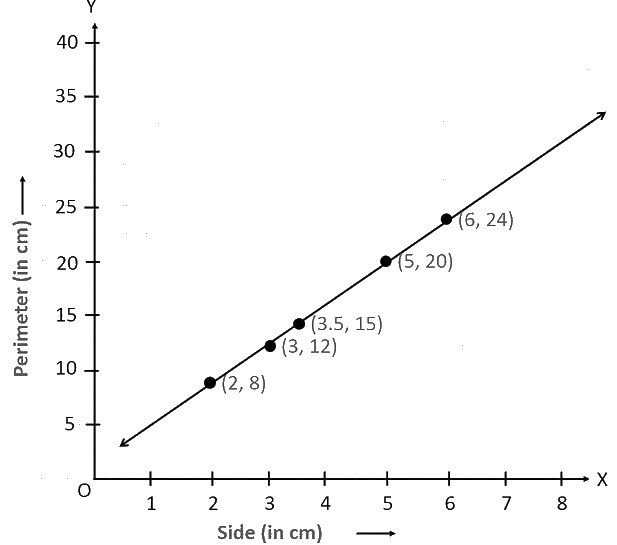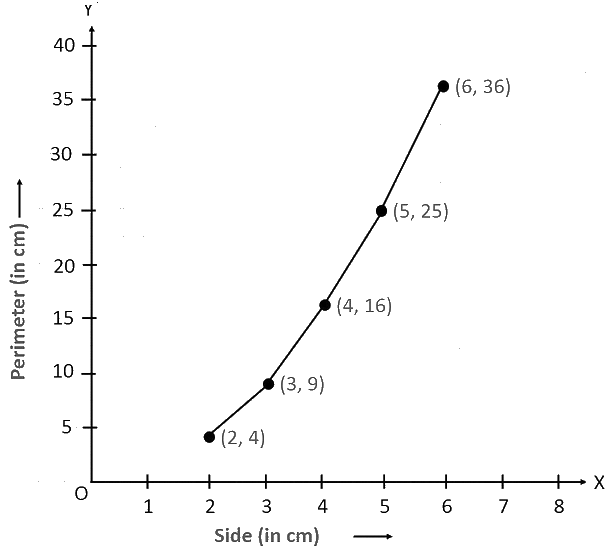# Ex.15.3 Q2 Introduction to Graphs Solutions - NCERT Maths Class 8

Go back to  'Ex.15.3'

## Question

Draw a graph for the following.

(i)

 Side of square (in $$\rm{cm}$$) $$2$$ $$3$$ $$3.5$$ $$5$$ $$6$$ Perimeter (in $$\rm{cm}$$) $$8$$ $$12$$ $$14$$ $$20$$ $$24$$

Is it a linear graph?

(ii)

 Side of square (in $$\rm{cm}$$) $$2$$ $$3$$ $$4$$ $$5$$ $$6$$ Area (in $$\rm{cm}^2$$) $$4$$ $$9$$ $$16$$ $$25$$ $$36$$

Is it a linear graph?

## Text Solution

Reasoning:

A line graph which is a whole unbroken line is called a linear graph.

Steps:

(i) Choosing a suitable scale,

For $$x$$-axis, $$2$$ unit $$= 1\, \rm{cm}$$ and for $$y$$-axis, $$2$$ unit $$= 5\, \rm{cm}$$

We can represent the side of a square on $$x$$-axis and the perimeter of that square on $$y$$-axis. A graph of the given data is drawn as follows.It is a linear graph.

(ii) Choosing a suitable scale,

For x-axis, $$2$$ unit $$= 1 \,\rm{cm}$$ and for $$y$$-axis, $$2$$ unit $$= 5\;\rm{cm}$$

We can represent the side of a square on the x-axis and the area of that square on $$y$$-axis. A graph of the given data is as follows.It is not a linear graph.

Learn from the best math teachers and top your exams

• Live one on one classroom and doubt clearing
• Practice worksheets in and after class for conceptual clarity
• Personalized curriculum to keep up with school